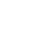# Python编程语言控制语句流程结构-循环语句允（Iterator）

Python编程语言提供以下类型的循环来处理循环需求。

while循环

for循环

Python支持以下控制语句。

break语句

continue语句

pass语句

String，List或Tuple对象可用于创建Iterator。

``````list = [1,2,3,4]
it = iter(list) # this builds an iterator object
print (next(it)) #prints next available element in iterator
# Iterator object can be traversed using regular for statement
for x in it:
print (x, end=" ")
or using next() function
while True:
try:
print (next(it))
except StopIteration:
sys.exit() #you have to import sys module for this
``````

``````#!usr/bin/python3
import sys
def fibonacci(n): #generator function
a, b, counter = 0, 1, 0
while True:
if (counter > n):
return
yield a
a, b = b, a + b
counter += 1
f = fibonacci(5) #f is iterator object
while True:
try:
print (next(f), end=" ")
except StopIteration:
sys.exit()
``````

《Python编程语言控制语句流程结构-循环语句允（Iterator）》